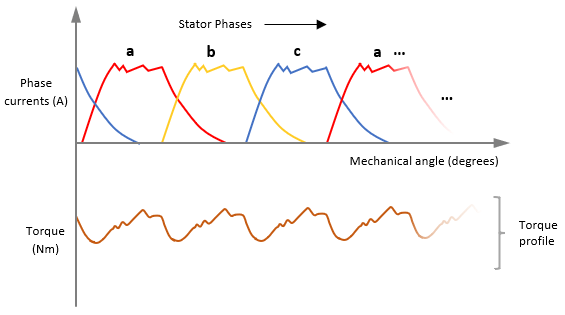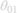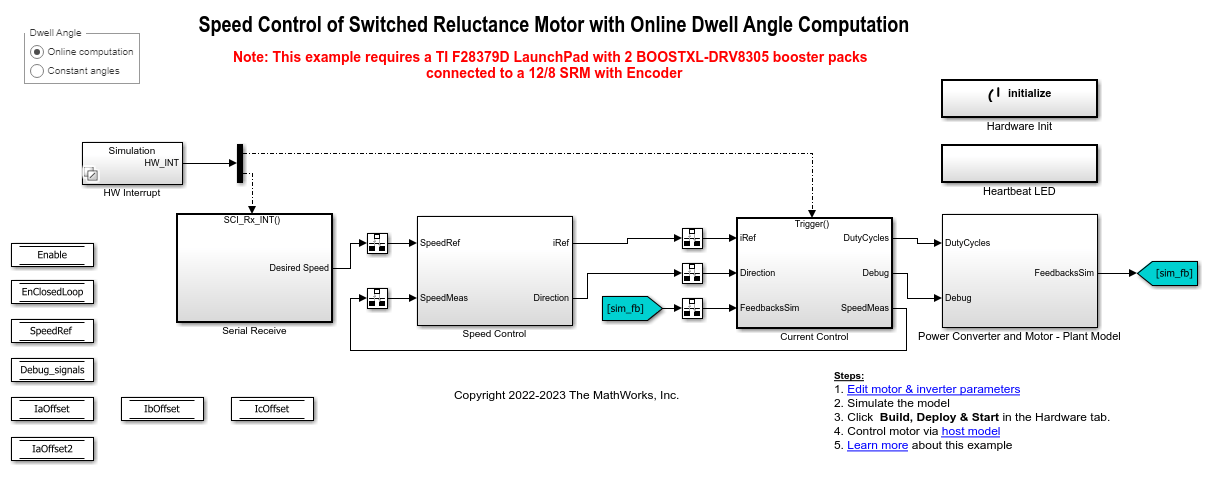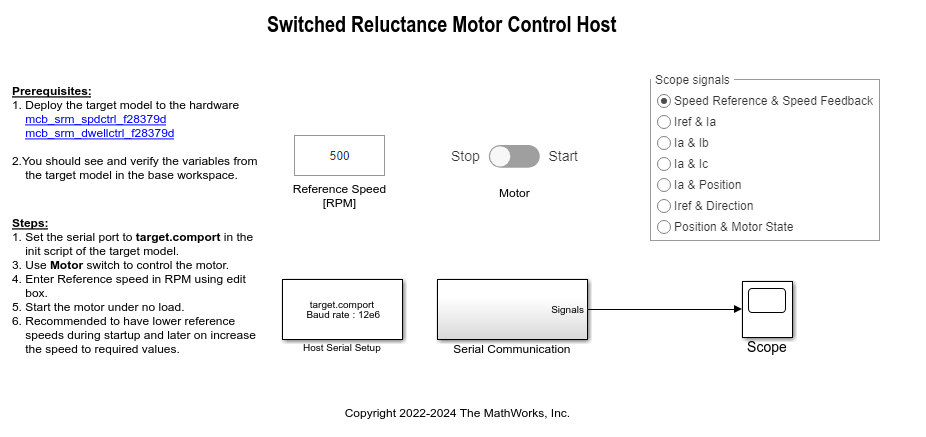# Dwell Angle Computation for SRM Speed Control

This example computes the dwell angle of a 12/8 Switched Reluctance Motor (SRM) online and uses it with a delta controller to control the motor speed.

SRMs need commutation switching sequences to run. These sequences determine when the motor phases can be switched On and Off, and therefore, run the motor while controlling the motor speed. Each commutation switching sequence (which forms a pulse train) can be used to control (turn On or Off) the corresponding motor phase. The activation period defined by the turn On () and turn Off () angles is known as the dwell angle.

Dwell angle is one of the control variables for the motor. The optimal dwell angle depends on the motor parameters and the control objective.

A large dwell angle may result in overlapped phase current pulses, which results in simultaneous activation of adjacent phases leading to greater power consumption. This enhances motor performance by reducing torque ripples as shown below. However, a larger dwell angle makes the motor less energy efficient.A small dwell angle leads to a reduced overlap of phase current pulses. This causes the motor to draw less power, and therefore, become more energy efficient. However, this usually results in larger torque ripples as shown below, which may impact motor performance.This example computes an optimum dwell angle such that it achieves a balance between motor energy efficiency and motor torque ripple amplitude. For a given reference speed and load condition, the example computes the optimum dwell angle using only a few SRM design parameters.

The following image shows an example of torque profile corresponding to an optimized dwell angle.The example computes the dwell angle using the anglesand, as shown below.is the angle measured between phase activation and the point when the initial rise in phase current ends.is the angle measured between phase shutdown and the point when the phase current is zero. For a given phase, usually>due to the rising inductance profile in an SRM.

Note: The example computesandonly for phase A, and assumes that all the phases behave identically.

The example model uses a PI controller (for speed control) and a delta controller (for current control), along with an online dwell angle computation algorithm to optimize performance of the SRM. The example uses an easy-to-implement, low-runtime, and computationally inexpensive delta controller for current control. The current control loop runs at 50KHz (20µs). In addition, the example uses the necessary filters to enable smoother transitions forand.

Note: Using online dwell angle computation to control SRM speed may reduce the range of achievable torque and speed.

Alternatively, you may use this example to run the SRM with delta current controller using a fixed dwell angle by specifyingandin the model initialization script.

### Model

The example includes the model mcb_srm_dwellctrl_f28379d.

You can use the model for both simulation and code generation. To open a Simulink® model, you can also use the open_system command at the MATLAB® command prompt. For example, use this command for the F28379D based controller:

open_system('mcb_srm_dwellctrl_f28379d.slx');For details about the supported hardware configuration, see Required Hardware in the Generate Code and Deploy Model to Target Hardware section.

### Required MathWorks® Products

To simulate model:

• Motor Control Blockset™

• Simscape™ Electrical™

To generate code and deploy model:

• Motor Control Blockset™

• Fixed-Point Designer™ (only needed for optimized code generation)

• Embedded Coder®

• C2000™ Microcontroller Blockset

### Prerequisites

Obtain the motor parameters. The example model includes default SRM parameters. If required, you can replace them with values from a motor datasheet or other sources.

Update SRM parameters in the model initialization script associated with the Simulink model. For instructions, see Estimate Control Gains and Use Utility Functions.

If required, update the following parameters in the model initialization script:

• PWM_frequency — Enter the switching frequency of PWM. Note that you may need to tune controller gains again if you change this value.

• Kp and Ki — Enter the PI controller gains. Note that the example does not automatically compute these values. Set these gains according to the hardware setup.

• thetaON — Enter the motor phase turn-on electrical position at which the switching sequence turns 0 to 1 and energizes the corresponding phase. The model uses this value only when you set the Dwell Angle button in the target model to Constant angles.

• thetaOFF — Enter the motor phase turn-off electrical position at which the switching sequence turns 1 to 0 and de-energizes the corresponding phase. The model uses this value only when you set Dwell Angle button in the target model to Constant angles.

Note: The example supports only single data type and per-unit computation.

### Simulate Model

This example supports simulation. Follow these steps to simulate the model.

1. Open the target model included with this example.

2. Click Run on the Simulation tab to simulate the model.

3. Click Data Inspector on the Simulation tab to view and analyze the simulation results.

### Generate Code and Deploy Model to Target Hardware

This section shows how to generate code and run the control algorithm on the target hardware.

This example uses a host and a target model. The host model is a user interface to the controller hardware board. You can run the host model on the host computer. The prerequisite to use the host model is to deploy the target model to the controller hardware board. The host model uses serial communication to command the target Simulink model and run the motor in a closed-loop control.

Required Hardware

The example supports the following hardware configuration. You can also use the target model name to open the model from the MATLAB command prompt.

The example uses the ADC current sensors available in the inverter boards to measure the 3 phase motor currents needed for controlling SRM.

Hardware Connections

The example requires 2 BOOSTXL-DRV8305 inverters connected in the following configuration.Generate Code and Run Model on Target Hardware

1. Simulate the target model and observe the simulation results.

2. Complete the hardware connections.

3. The model computes the ADC (or current) offset values by default. To disable this functionality, update the value 0 to the variable inverter.ADCOffsetCalibEnable in the model initialization script.

Alternatively, you can compute the ADC offset values and update them manually in the model initialization script.

4. Open the target model. If you want to change the default hardware configuration settings for the model, see Model Configuration Parameters.

5. Load a sample program to CPU2 of LAUNCHXL-F28379D. For example, you can use the program that operates the CPU2 blue LED by using GPIO31 (c28379D_cpu2_blink.slx) and ensure that CPU2 is not mistakenly configured to use the board peripherals intended for CPU1.

6. Use the Dwell Angle button to select one of the following options:

• Online computation — Select this option to enable online dwell angle computation algorithm and use the dwell angle computed in real-time for speed control of SRM.

• Constant angles — Select this option to disable online dwell angle computation and use the variables thetaON and thetaOFF (that define a fixed dwell angle) in the model initialization script for speed control of SRM.

7. Click Build, Deploy & Start on the Hardware tab to deploy the target model to the hardware.

8. Observe and verify the variables populated by the target model in the base workspace.

9. Click the host model hyperlink in the target model to open the associated host model. You can also use the open_system command to open the host model. Use this command for a F28379D based controller.

open_system('mcb_srm_spdctrl_host.slx');For details about the serial communication between the host and target models, see Host-Target Communication.

10. In the model initialization script associated with the target model, specify the communication port using the variable target.comport. This variable updates the Port parameter of the Host Serial Setup, Host Serial Receive, and Host Serial Transmit blocks available in the host model.

11. Update the reference speed value in the Reference Speed (RPM) field in the host model. We recommend that you enter lower speeds initially during motor start up and gradually increase the speed later.

12. In the host model, select the debug signals that you want to monitor.

13. Click Run on the Simulation tab to run the host model.

14. After you connect the load to the motor shaft, change the position of the Motor switch to Start, to start running the motor.

If the motor does not align or if it fails to start running in open-loop mode, increase the values of the start-up parameters listed in the Motor Start Up Parameters section of the model initialization script.

15. The example performs the following procedures:

• Calibrate the quadrature encoder sensor (which includes alignment of the rotor with stator phase A).

• Use the fixed dwell angle defined in the model initialization script to run SRM in closed loop according to Reference Speed (RPM).

OR

Compute optimal dwell angle according to the load conditions and uses it to run SRM in closed loop according to Reference Speed (RPM).

16. Observe the debug signals received from target in the Scope available in the host model.

### References

 C. Mademlis and I. Kioskeridis, "Performance optimization in switched reluctance motor drives with online commutation angle control," in IEEE Transactions on Energy Conversion, vol. 18, no. 3, pp. 448-457, Sept. 2003, doi: 10.1109/TEC.2003.815854.